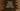# Python program to convert meter to yard## meter-yard conversion in Python :

This tutorial will show you how to convert a distance of meter to yard. If you are getting the value in one unit, you need to convert it to a different unit if your program is working on a separate unit. This is a commonly faced problem. If you are using any third-party APIs and if that API returns data in meter, you need to convert it to yard if you are showing the information in yard to the user.

This is a beginner level python program and it will show you how to convert a value to yard if it is available in meter. You can use the inverted method to convert a yard value to meter.

## Explanation :

The yard conversion of 1 meter is 1.09361. So, for x meter, the yard will be x multiplied by 1.09361. Our program will take the value of meter as an input from the user. It will then convert the value to yard and print it to the user.

### Python program :

``````meter_to_yard = 1.09361

m = float(input("Enter the meter value : "))
y = m * meter_to_yard

print("{} meter = {} yard".format(m,y))``````

### Sample output :

``````Enter the meter value : 100
100.0 meter = 109.36099999999999 yard

Enter the meter value : 211
211.0 meter = 230.75171 yard``````